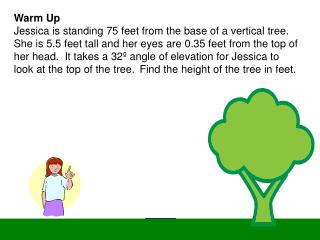# Warm Up - PowerPoint PPT PresentationDownload PresentationWarm Up

Warm UpDownload Presentation## Warm Up

- - - - - - - - - - - - - - - - - - - - - - - - - - - E N D - - - - - - - - - - - - - - - - - - - - - - - - - - -
##### Presentation Transcript

1. Warm Up Jessica is standing 75 feet from the base of a vertical tree. She is 5.5 feet tall and her eyes are 0.35 feet from the top of her head. It takes a 32º angle of elevation for Jessica to look at the top of the tree. Find the height of the tree in feet.

2. Answer Height of Jessica’s eyes 5.5 – 0.35 = 5.15 x 32° 5.15 ft 75 ft cos32 = 75 x x = 88.43838025 Tree height 88.43838025 + 5.15 = 93.58838025 feet The height of the tree is 94 feet.

3. Trig Game Plan Date: 10/7/13

4. Bearing Word Problems Bearing Word Problems are an application of trigonometry that is used in navigation. Caution: Angles in Bearing Problems are not measured from standard position.

5. Bearing There are two methods for expressing bearing. When a single angle is given, such as 164˚, it is understood that the bearing is measured in a clockwise direction from due north.

6. Bearing The second method for expressing bearing starts with a North-South line and uses an acute angle to show the direction, either East or West, from this line.

7. Radar stations A and B are on an east-west line, 8.6 km apart. Station A detects a plane at C, on a bearing of 53˚. Stations B simultaneously detects the same plane, on a bearing of 323˚. Find the distance from B to C. Example 1a: SOLVING A PROBLEM INVOLVING BEARING (FIRST METHOD) A line drawn due north is perpendicular to an east-west line, so right angles are formed at A and B, and angles CAB and CBA can be found as shown in the figure. ∠C is a right angle because ∠CAB and ∠CBA are complementary.

8. Find distance a by using the cosine function for angle A. Example 1a The distance from B to C is about 5.2 km.

9. Example 1b SOLVING A PROBLEM INVOLVING BEARING (FIRST METHOD) Radar stations A and B are on an east-west line, 3.7 km apart. Station A detects a plane at C, on a bearing on 61˚. Station B simultaneously detects the same plane, on a bearing of 331˚. Find the distance from A to C.

10. Caution A correctly labeled sketch is crucial when solving bearing applications. Some of the necessary information is often not directly stated in the problem and can be determined only from the sketch.

11. Example 2a: SOLVING PROBLEM INVOLVING BEARING (SECOND METHOD) The bearing from A to C is S 52˚E. The bearing from A to B is N 84˚E. The bearing from B to C is S 38˚W. A plane flying at 250 mph takes 2.4 hours to go from A to B. Find the distance from A to C. To draw the sketch, first draw the two bearings from point A. Choose a point B on the bearing N 84˚ E from A, and draw the bearing to C, which is located at the intersection of the bearing lines from A and B.

12. Example 2a SOLVING PROBLEM INVOLVING BEARING (SECOND METHOD) (cont.) The distance from A to B is about 430 miles.

13. Example 2b The information in the example gives and The bearing from A to C is N 64˚W. The bearing from A to B is S 82˚W. The bearing from B to C is N 26˚E. A plane flying at 350 mph take 1.8 hours to go from A to B. Find the distance from B to C.

14. Example 2b SOLVING PROBLEM INVOLVING BEARING (SECOND METHOD) The sum of the measures of angles EAB and FBA is 180º because they are interior angles on the same side of a transversal. 90° 34° 56° 98°

15. Example 2b 630 mi SOLVING PROBLEM INVOLVING BEARING (SECOND METHOD) It takes 1.8 hours at 350 mph to fly from A to B, so 90° 34° 56° 98° The distance from B to C is about 350 miles.